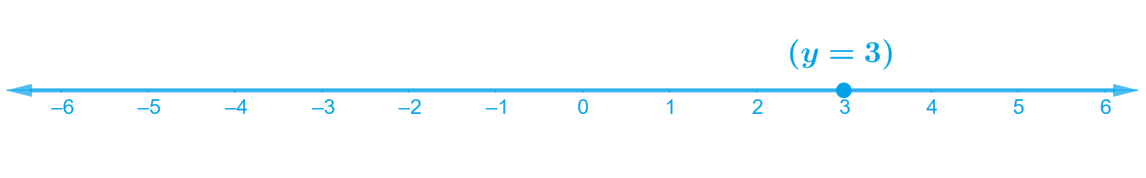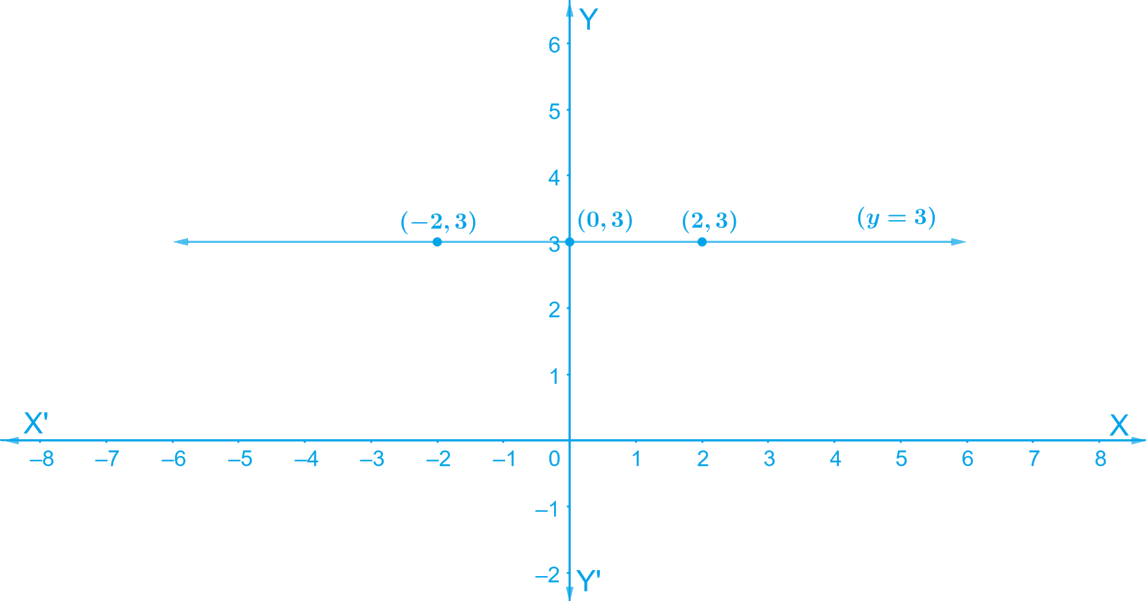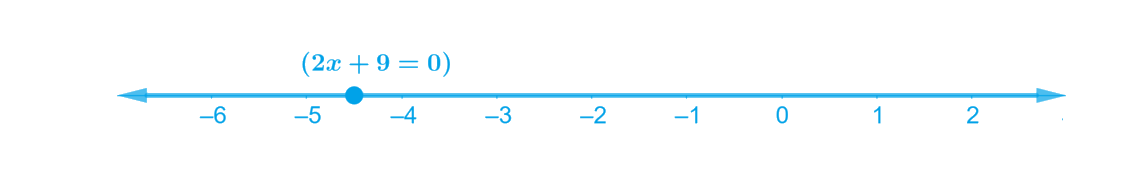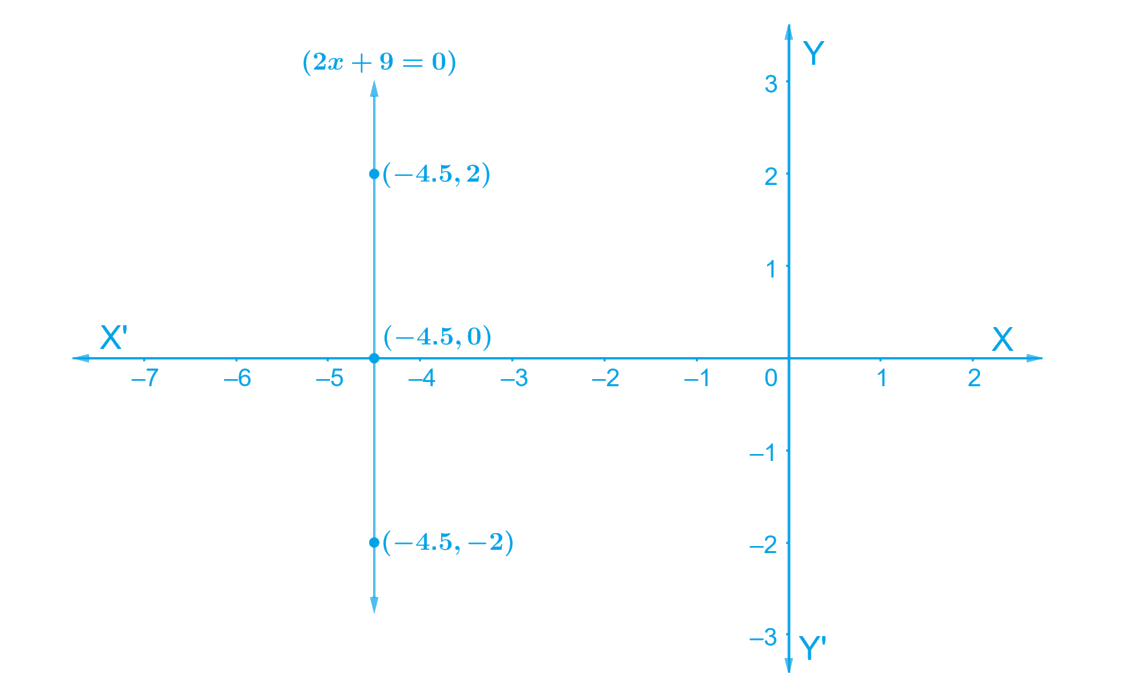# Exercise E4.4 Linear Equations in Two Variables NCERT Solutions Class 9

Go back to  'Linear Equations in Two Variables'

## Chapter 4 Ex.4.4 Question 1

Give the geometric representation of $$y = 3$$ as an equation

(i) in one variable

(ii) in two variables

#### Steps:

(i) Given,

Considering $$y = 3$$ is the equation in one variable

The representation of the solution on the number when $$y = 3$$ is treated as an equation in one variable

In one variable, $$y = 3$$ represents a point as shown in following figure.(ii) Given:

Considering $$y = 3$$ is the equation in two variables

We know that $$y = 3$$ can be written as $$0.x + y = 0$$.

In two variables, $$y = 3$$ represents a straight line passing through point $$(0, 3)$$ and parallel to $$x$$-axis. It is a collection of all the points on the plane, having their $$y$$-coordinate as $$3.$$

Hence,

• When, $$x = 0$$, we get $$y = 3$$;
• When $$x = 2$$, we get $$y = 3$$;
• When $$x = -2$$, we get $$y = 3$$ are the solutions for the equations.

Plotting the points $$(0, 3) (2, 3)$$ and $$(–2, 3)$$ and on joining them we get the graph $$AB$$ as a line parallel to $$x$$-axis at a distance of $$3\,\rm units$$ above it

The graphical representation is shown below:## Chapter 4 Ex.4.4 Question 2

Give the geometric representations of

$$2x + 9 = 0$$ as an equation

(i) in one variable

(ii) in two variables

#### Steps:

(i) Given: $$2x + 9 = 0$$ is the Linear Equation -------- Equation (1)

\begin{align} 2 x + 9 &= 0 \\ 2 x &= - 9 \\ x &= \frac { - 9 } { 2 } \\ &= -4.5 \end{align}

Hence, in one variable $$2x + 9 = 0$$ represents a point as shown in the following figure.(ii) Given: $$2x + 9 = 0$$ is the Linear Equation ------- Equation (1)

We know that $$2x + 9 = 0$$ can be written as $$2x + 0y + 9 = 0$$ as a linear equation in variables $$x$$ and $$y$$.

Value of $$y$$ is always $$0$$. However, $$x$$ must satisfy the relation $$2x + 9= 0$$

\begin{align} \text { i.e. } \;\;\; x &= \frac { - 9 } { 2 } \\ & = - 4.5 \end{align}

Hence,

• When, $$y= 0$$, we get $$x= -4.5$$;
• When $$y = 2$$, we get $$x= -4.5$$;
• When $$y = - 2$$, we get $$x= -4.5$$ are the solutions for the equations.

Hence three solution of the given equation are,

$$y = 0$$; $$y = 2$$ and $$y =\, – 2$$.

Therefore, plotting the point and on joining them we get the graph $$AB$$ as a line parallel to $$y$$-axis at a distance of on the left of $$y$$-axis It is a collection of all points of the plane, having their $$x$$-coordinate as $$4.5$$Related Sections
Related Sections
Instant doubt clearing with Cuemath Advanced Math Program